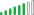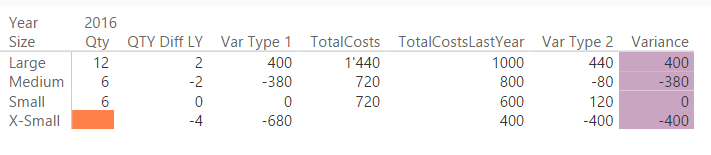cancel
Showing results for
Did you mean:Helper II

## Variance calculations at a row level - SUMX not working argh!

Hi all,

This has been my achiles heel with all things power and if I, aka you guys, find a solution then it will revolutionise my world!

Very simple example, calculating a quantity variance - formula is (Quantity TY - Quantity LY) * Price LY, but when no products were sold TY but we did sell some LY then I want Total Cost TY - Total Cost LY.  But SUMX doesn't work because there is no 'TY event'.

My work around is to use power query which produces a table that covers off every scenario that has ever happened and if there is 'no event' i.e. nothing sold then the power query would enter a 0 which would then 'trigger' the SUMX to work.  However, I am dealing with a large data set with many differnet scenarios i.e. TY Actual, Forecast, Previous Forecast, Budget, LY Actual and so power query then takes over an hour to refresh.

The table of data is below, I want for Jul-16, X-Small Jumper to return -400 i.e. (0-4)*100 and my SUMX formual is below the table, I need to include CALCULATETABLE and SUMMARIZE functions because the month, product, size fields are all from link tables.

 Mth Product Size Qty Cost Jul-15 Jumper Large 10 1000 Jul-15 Jumper Medium 8 800 Jul-15 Jumper Small 6 600 Jul-15 Jumper X-Small 4 400 Jul-16 Jumper Large 12 1000 Jul-16 Jumper Medium 6 800 Jul-16 Jumper Small 6 600

QuantityVar:=SUMX(

CALCULATETABLE(

SUMMARIZE('Table1',Table2[Mth],Table3[Product],Table4[Size])),

IF(OR([Quantity]=0,ISBLANK([Quantity])),[Cost\$]-[Cost\$LY],

([Quantity]-[QuantityLY])*[Price\$LY]))

3 REPLIES 3Microsoft

Hi @Dan80,

Could you please post raw data of tables and formulas of measures([Cost\$],[Cost\$LY],etc.)? And how about using the following formula to calculate QuantityVar?

NewTable= CALCULATETABLE(
SUMMARIZE('Table1',Table2[Mth],Table3[Product],Table4[Size]))

QuantityVar = IF([Quantity]=BLANK(),SUMX(NewTable, [Cost\$]-[Cost\$LY]),
SUMX(NewTable, ([Quantity]-[QuantityLY])*[Price\$LY]))

Thanks,
Lydia Zhang

Community Support Team _ Lydia Zhang
If this post helps, then please consider Accept it as the solution to help the other members find it more quickly.Solution Supplier

Hi Dan

I tried to make a sample with your info provided. Not all the details are clear to me I must say.

I used your data provided but changed the cost values as your values seem to be a multiplication of qty * cost per item. Furthermore, where is the price value stored? I added a price column in the data table, which looks like this (please don't get confused with the German dates):I set the following relations, is this setup correct?Next I created some measures.

- QTY Diff LY: Calculates the difference in qty compared to last year

`QTY Diff LY = SUM(Table1[Qty])- CALCULATE(SUM(Table1[Qty]),SAMEPERIODLASTYEAR(Table2[Date]))`

The key here is to use the SAMEPERIODLASTYEAR function

- Var Type 1: Calculates the variance in case you have sales in both years. This is referencing the QTL Diff LY measure

```Var Type 1 =
[QTY Diff LY]
* CALCULATE(
SUM(Table1[Price]),SAMEPERIODLASTYEAR(Table2[Date])
)```

- TotalCosts

`TotalCosts = SUM(Table1[Cost]) * SUM(Table1[Qty])`

- TotalCostsLastYear

```TotalCostsLastYear = CALCULATE(
[TotalCosts],SAMEPERIODLASTYEAR(Table2[Date]))```

- Var Type 2: Calculates the variance in case you have not sales this year

`Var Type 2 = [TotalCosts] - [TotalCostsLastYear]`

- Variance: simple if that decides wheter to use type 1 or 2

`Variance = IF(SUM(Table1[Qty]) > 0; [Var Type 1]; [Var Type 2_a])`

Put it all in a matrix it looks like this:Does this make sense?

This is by far not a complete solution but I hope it leads you in the right direction. And of course you do not need that many maesures, you actually could combine it all in a single measure.

One question is left for me: How is your calculation in case you don't have sales in the last year? Where do you take the price from?

Hope this helps

JJHelper II

Unfortunatley the calculation needs to rely on a SUMX formula otherwise the sum of all the results won't match the total in the pivot.  The other issue is that depending on how the data is 'sliced and diced' the total will change.  These scenarios are difficult using the example I gave as it is a very simple data set.  I have a feeling that this is the first time ever that I have come unstuck with 'power'.Announcements#### Exclusive opportunity for Women!

Join us for a free, hands-on Microsoft workshop led by women trainers for women where you will learn how to build a Dashboard in a Day!#### Power Platform Conference-Power BI and Fabric Sessions

Join us Oct 1 - 6 in Las Vegas for the Microsoft Power Platform Conference.Top Solution Authors
Top Kudoed Authors
Users online (1,794)